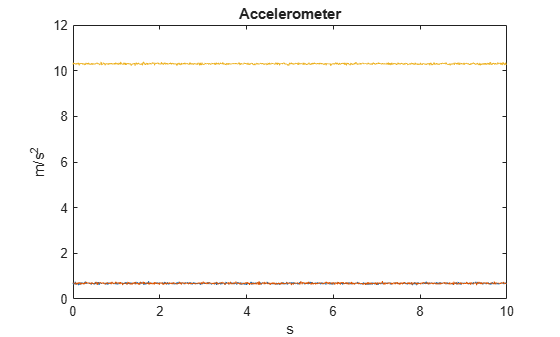accelparams

Accelerometer sensor parameters

Description

The accelparams class creates an accelerometer sensor parameters object. You can use this object to model an accelerometer when simulating an IMU with imuSensor. See the Algorithms section of imuSensor for details of accelparams modeling.

Creation

Description

params = accelparams returns an ideal accelerometer sensor parameters object with default values.

example

params = accelparams(Name,Value) configures an accelerometer sensor parameters object properties using one or more Name-Value pair arguments. Name is a property name and Value is the corresponding value. Name must appear inside single quotes (''). You can specify several name-value pair arguments in any order as (Name1,Value1,...,NameN,ValueN). Any unspecified properties take default values.

Properties

expand all

Maximum sensor reading in m/s2, specified as a real positive scalar.

Data Types: single | double

Resolution of sensor measurements in (m/s2)/LSB, specified as a real nonnegative scalar. Here, LSB is the acronym for least significant bit. Resolution is often referred as Scale Factor for accelerometer.

Data Types: single | double

Constant sensor offset bias in m/s2, specified as a real scalar or 3-element row vector. Any scalar input is converted into a real 3-element row vector where each element has the input scalar value.

Data Types: single | double

Sensor axes skew in percentage, specified as a scalar, a 3-element row vector, or a 3-by-3 matrix with values ranging from 0 to 100. The diagonal elements of the matrix account for the misalignment effects for each axes. The off-diagonal elements account for the cross-axes misalignment effects. The measured state vmeasure is obtained from the true state vmeasure via the misalignment matrix as:

${v}_{measure}=\frac{1}{100}M\text{ }{v}_{true}=\frac{1}{100}\left[\begin{array}{ccc}{m}_{11}& {m}_{12}& {m}_{13}\\ {m}_{21}& {m}_{22}& {m}_{23}\\ {m}_{31}& {m}_{32}& {m}_{33}\end{array}\right]{v}_{true}$

• If you specify the property as a scalar, then all the off-diagonal elements of the matrix take the value of the specified scalar and all the diagonal elements are 100.

• If you specify the property as a vector [a b c], then m21 = m31 = a, m12 = m32 = b, and m13 = m23 = c. All the diagonal elements are 100.

Data Types: single | double

Power spectral density of sensor noise in (m/s2/√Hz), specified as a real scalar or 3-element row vector. This property corresponds to the velocity random walk (VRW). Any scalar input is converted into a real 3-element row vector where each element has the input scalar value.

Data Types: single | double

Instability of the bias offset in m/s2, specified as a real scalar or 3-element row vector. Any scalar input is converted into a real 3-element row vector where each element has the input scalar value.

Data Types: single | double

Integrated white noise of sensor in (m/s2)(√Hz), specified as a real scalar or 3-element row vector. Any scalar input is converted into a real 3-element row vector where each element has the input scalar value.

Data Types: single | double

Sensor bias from temperature in (m/s2)/℃, specified as a real scalar or 3-element row vector. Any scalar input is converted into a real 3-element row vector where each element has the input scalar value.

Data Types: single | double

Scale factor error from temperature in %/℃, specified as a real scalar or real 3-element row vector with values ranging from 0 to 100. Any scalar input is converted into a real 3-element row vector where each element has the input scalar value.

Data Types: single | double

Examples

collapse all

Generate accelerometer data for an imuSensor object from stationary inputs.

Generate an accelerometer parameter object with a maximum sensor reading of 19.6 $\mathrm{m}/{\mathrm{s}}^{2}$ and a resolution of 0.598 $\left(\mathrm{mm}/{\mathrm{s}}^{2}\right)/\mathrm{LSB}$. The constant offset bias is 0.49 $m/{s}^{2}$. The sensor has a power spectral density of 3920 $\left(\mu \mathrm{m}/{\mathrm{s}}^{2}\right)/\sqrt{\mathrm{Hz}}$. The bias from temperature is 0.294 $\left(\mathrm{m}/{\mathrm{s}}^{2}\right)$$/{}^{0}C$. The scale factor error from temperature is 0.02%$/{}^{0}C$. The sensor axes are skewed by 2%.

params = accelparams('MeasurementRange',19.6,'Resolution',0.598e-3,'ConstantBias',0.49,'NoiseDensity',3920e-6,'TemperatureBias',0.294,'TemperatureScaleFactor',0.02,'AxesMisalignment',2);

Use a sample rate of 100 Hz spaced out over 1000 samples. Create the imuSensor object using the accelerometer parameter object.

Fs = 100;
numSamples = 1000;
t = 0:1/Fs:(numSamples-1)/Fs;

imu = imuSensor('SampleRate', Fs, 'Accelerometer', params);

Generate accelerometer data from the imuSensor object.

orient = quaternion.ones(numSamples, 1);
acc = zeros(numSamples, 3);
angvel = zeros(numSamples, 3);

accelData = imu(acc, angvel, orient);

Plot the resultant accelerometer data.

plot(t, accelData)
title('Accelerometer')
xlabel('s')
ylabel('m/s^2')